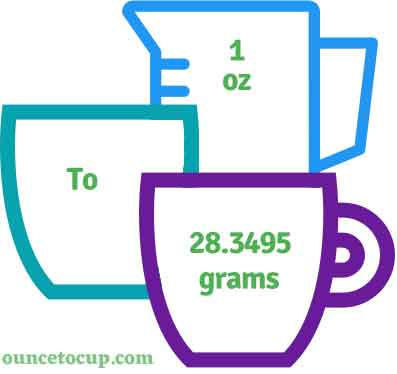# 6 Ounces to Grams (6 oz to g conversion)

Are you cooking your favorite dish? The detailed chart in the recipe includes the calculation of 6 ounces to g conversion? Do not worry; check this conversion tool to find how many 6 ounces equal to g in a minute. This 6 oz to g converter gives an exact measurement for any recipe you prepare.

Ounce Value:

oz

Gram Value:

g

6 Ounce = 170.097 Gram
(6 oz = 170.097 g)

Try our auto 6 ounce to gram calculator (Without Convert Button), Just change the first field value and you got final value.## How many grams is a 6 oz?

We know that the volume value of 6 oz is equal to 170.097 g. If you want to convert 6 fluid oz to an equal number of g, just multiply the volume value by 28.3495. Hence, 6 Ounce is equal to 170.097 g.

The Answer is: 6 US Fluid Ounces = 170.097 US Grams

6 oz = 170.097 g

Many of them try to search or find an answer for what is 6 ounces in g? So, we’ll start with 6 fl oz to g conversion to know how big is 6 oz.

## How To Calculate 6 fluid oz to g?

To calculate 6 fluid ounces to an equal number of gram, simply follow the steps below.

Fluid Ounces to Grams formula is:

Gram = Fluid Ounce * 28.3495

Assume that we are finding out how many g were found in 6 fl oz of water, multiply by 28.3495 to get the result.

Applying to Formula: gram = 6 oz * 28.3495 = 170.097 g.

## How To Convert 6 oz to g?

• To convert 6 fluid ounces to g,
• Simply multiply the 6 fluid ounce value by 28.3495.
• Applying to the formula, g = 6 ounces * 28.3495 [6x28.3495].
• Hence, 6 ounces is equal to 170.097 g.

## Some quick table references for ounce to gram conversions:

Ounce [oz]Gram [g]
1 oz28.3495 g
2 oz56.699 g
3 oz85.0485 g
4 oz113.398 g
5 oz141.7475 g
6 oz170.097 g
7 oz198.4465 g
8 oz226.796 g
9 oz255.1455 g
10 oz283.495 g
11 oz311.8445 g
12 oz340.194 g
13 oz368.5435 g
14 oz396.893 g
15 oz425.2425 g

## Reverse Calculation: How many ounces are in a 6 gram?

• To convert 6 gram to oz,
• Simply divide the 6 g by 28.3495.
• Then, applying the formula, ounce = 6 g / 28.3495 [6/28.3495 = 0.211644].
• Hence, 6 g is equal to 0.211644 oz.

### Related Converter:

Formula: Ounce to Grams

g = ounce * 28.3495

Applying to Formula,

g = 6*28.3495 = 170.097

6 oz = 170.097 g